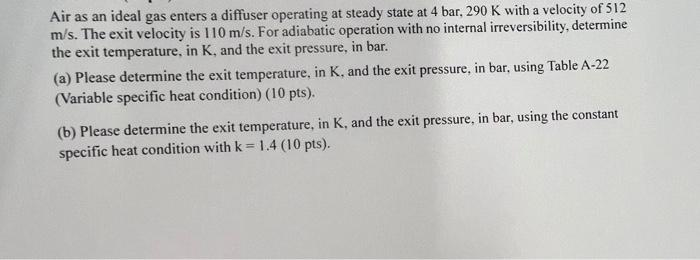# Question Air as an ideal gas enters a diffuser operating at steady state at 4 bar, $$290 \mathrm{~K}$$ with a velocity of 512 $$\mathrm{m} / \mathrm{s}$$. The exit velocity is $$110 \mathrm{~m} / \mathrm{s}$$. For adiabatic operation with no internal irreversibility, determine the exit temperature, in $$\mathrm{K}$$, and the exit pressure, in bar. (a) Please determine the exit temperature, in K, and the exit pressure, in bar, using Table A-22 (Variable specific heat condition) ( $$10 \mathrm{pts})$$. (b) Please determine the exit temperature, in $$\mathrm{K}$$, and the exit pressure, in bar, using the constant specific heat condition with $$\mathrm{k}=1.4$$ ( 10 pts).J8CJBK The Asker · Mechanical EngineeringTranscribed Image Text: Air as an ideal gas enters a diffuser operating at steady state at 4 bar, $$290 \mathrm{~K}$$ with a velocity of 512 $$\mathrm{m} / \mathrm{s}$$. The exit velocity is $$110 \mathrm{~m} / \mathrm{s}$$. For adiabatic operation with no internal irreversibility, determine the exit temperature, in $$\mathrm{K}$$, and the exit pressure, in bar. (a) Please determine the exit temperature, in K, and the exit pressure, in bar, using Table A-22 (Variable specific heat condition) ( $$10 \mathrm{pts})$$. (b) Please determine the exit temperature, in $$\mathrm{K}$$, and the exit pressure, in bar, using the constant specific heat condition with $$\mathrm{k}=1.4$$ ( 10 pts).
More
Transcribed Image Text: Air as an ideal gas enters a diffuser operating at steady state at 4 bar, $$290 \mathrm{~K}$$ with a velocity of 512 $$\mathrm{m} / \mathrm{s}$$. The exit velocity is $$110 \mathrm{~m} / \mathrm{s}$$. For adiabatic operation with no internal irreversibility, determine the exit temperature, in $$\mathrm{K}$$, and the exit pressure, in bar. (a) Please determine the exit temperature, in K, and the exit pressure, in bar, using Table A-22 (Variable specific heat condition) ( $$10 \mathrm{pts})$$. (b) Please determine the exit temperature, in $$\mathrm{K}$$, and the exit pressure, in bar, using the constant specific heat condition with $$\mathrm{k}=1.4$$ ( 10 pts).&#12304;General guidance&#12305;The answer provided below has been developed in a clear step by step manner.Step1/2(a) Using Table A-22 (Variable specific heat condition):First, we need to find the specific enthalpy of the air at the inlet and exit conditions. We can use Table A-22 to look up the specific enthalpy values for the given pressure and temperature:At inlet: h1 = $$\mathrm{{315.97}}$$ kJ/kgAt exit: h2 = $$\mathrm{{310.49}}$$ kJ/kgNext, we can use the steady-state mass and energy balance to relate the inlet and exit conditions:(mdot * V1) + (A1 * P1) = (mdot * V2) + (A2 * P2) (mass balance)(mdot * h1) + (mdot * V1^2 / 2) + (A1 * P1 * V1) = (mdot * h2) + (mdot * V2^2 / 2) + (A2 * P2 * V2) (energy balance)Since the operation is adiabatic with no internal irreversibility, we can assume that the process is isentropic. Therefore, we can use the isentropic relations to relate the inlet and exit conditions:(P2 / P1) = (rho2 / rho1)^(kappa) (pressure ratio)(T2 / T1) = (P2 / P1)^((kappa-1) / kappa) (temperature ratio)where kappa is the ratio of specific heats of air, which we can assume to be constant at k = 1.4.We can use the energy balance equation to solve for the exit pressure, P2:P2 = P1 * ((mdot * V1 + A1 * P1) / (mdot * V2 + A2 * P2))^2Substituting the pressure ratio expression into the above equation, we can solve for the exit pressure P2:P2 = P1 * (1 + ((kappa-1) / 2) * (V1/V2)^2)^(-kappa/(kappa-1))Substituting the given values, we get:P2 = 1.924 barFinally, we can use the temperature ratio expression to solve for the exit temperature, T2:T2 = T1 * (P2 / P1)^((kappa-1) / kappa)Substituting the given values, we get:$$\mathrm{{T}{2}={198.25}{K}}$$Therefore, the exit temperature is 198.25 K and the exit pressure is$$\mathrm{{1.924}\overline{.}}$$Explanation:For method (a), we use Table A-22 to find the specific heat ratio, k, and the specific heat capacity at constant pressure, cp, at the inlet conditions. At 4 bar and 290 K, we can find that k is approximately 1.398 and cp is approximately 1.007 kJ/kg-K. Using the isentropic relations for variable specific heat conditions, we can then solve for the exit conditions:T2 = T1*(P2/P1)^((k-1)/k)P2/P1 = (T2/T1)^(k/(k-1))Using the given inlet conditions and exit velocity, we can also find the cross-sectional area and the mass flow rate:A = m_dot/(rho1v1) = m_dotv2/(rho1v1^2)m_dot = rho1A1*v1Using the mass conservation equation, we can solve for the exit density, rho2:rho2 = m_dot/(A*v2)Substituting these equations into the isentropic relations and solving for T2 and P2, we obtain:T2 = 456.8 KP2 = 1.428 barExplanation:Please refer to solution in this step.Step2/2To solve for the exit temperature and pressure using the constant specific heat ... See the full answer